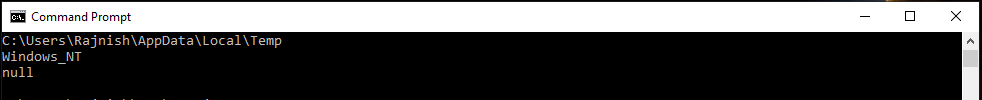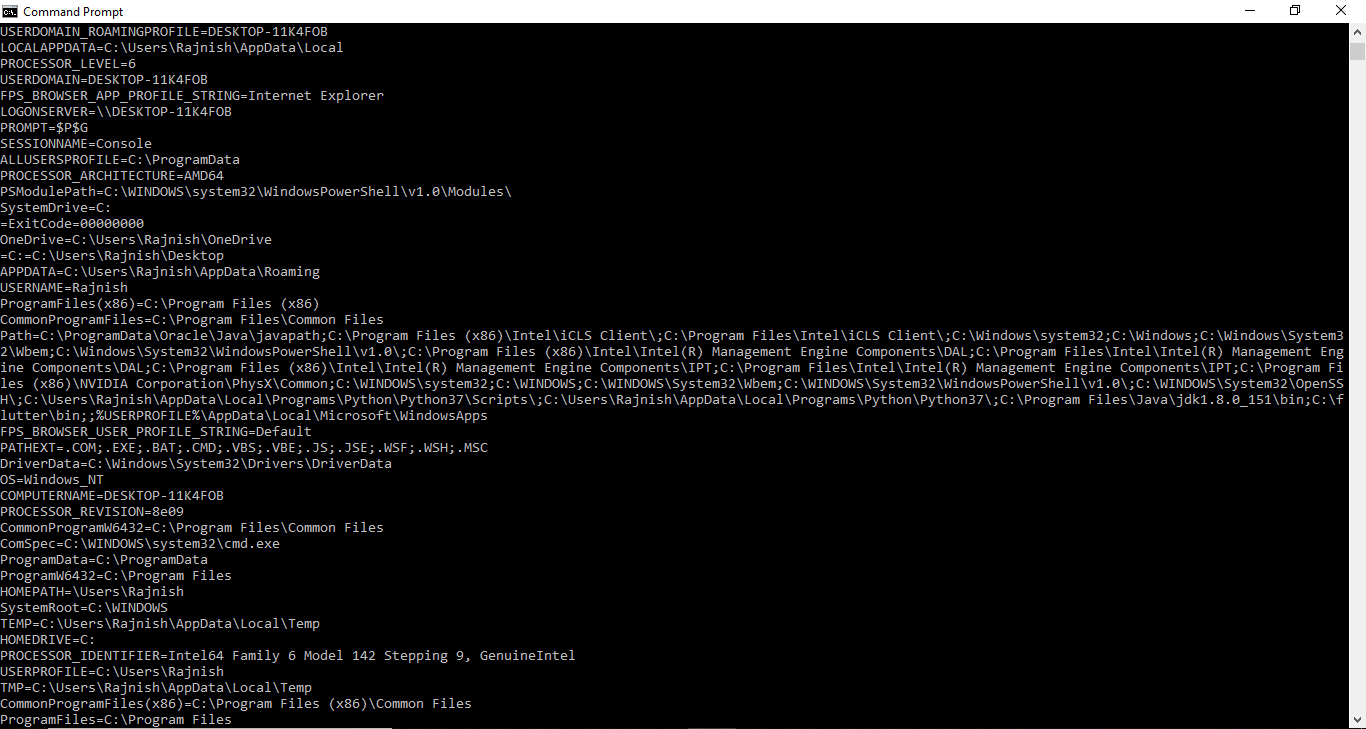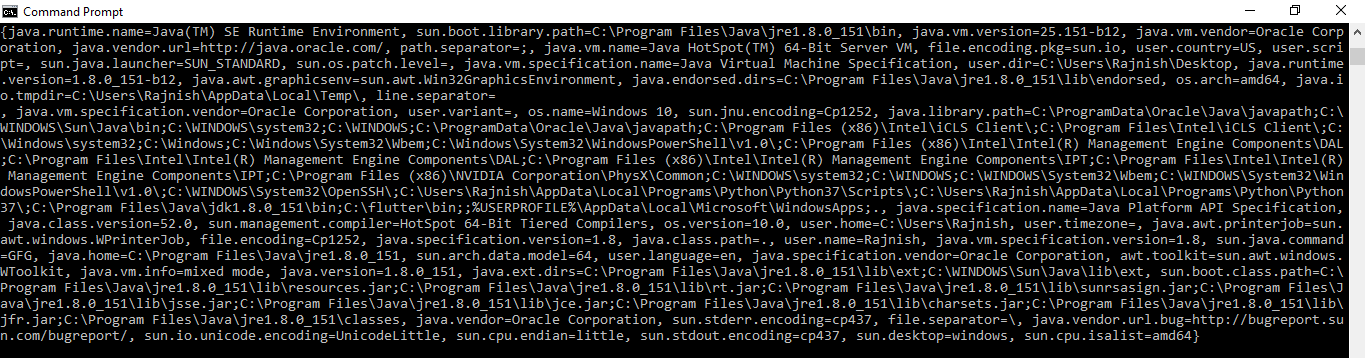# How to get the value of System Property and Environment Variable in Java?

### How to get the value of Environment variables?

The System class in Java provides a method named System.getenv() which can be used to get the value of an environment variable set in the current system.

Syntax:

```public static String getenv(String key);

where key is the Environment variable
whose values we want
```

Below example illustrates how to use System.getenv() to get the System environment variable:

Example 1: To get the value of a specific environment variable

 `// Java program to get the value ` `// of a specific environment variable ` `// using System.getenv() method ` ` `  `public` `class` `GFG { ` `    ``public` `static` `void` `main(String[] args) ` `    ``{ ` ` `  `        ``// Get the value of ` `        ``// the TEMP environment variable ` `        ``System.out.println(System.getenv(``"TEMP"``)); ` ` `  `        ``// Get the value of ` `        ``// the OS environment variable ` `        ``System.out.println(System.getenv(``"OS"``)); ` ` `  `        ``// Get the value of ` `        ``// the JAVA_HOME environment variable ` `        ``System.out.println(System.getenv(``"JAVA_HOME"``)); ` `    ``} ` `} `

Output:Example 2: To get the value of all environment variables at once

 `// Java program to get the value ` `// of all environment variables at once ` `// using System.getenv() method ` ` `  `import` `java.util.Map; ` ` `  `public` `class` `GFG { ` `    ``public` `static` `void` `main(String[] args) ` `    ``{ ` ` `  `        ``// Get the value of ` `        ``// all environment variables at once ` `        ``// and store it in Map ` `        ``Map env ` `            ``= System.getenv(); ` ` `  `        ``for` `(String envName : env.keySet()) { ` `            ``System.out.format(``"%s=%s%n"``, ` `                              ``envName, ` `                              ``env.get(envName)); ` `        ``} ` `    ``} ` `} `

Output:Note: The output will depend on the system on which you run the above code. A sample output is given above

### How to get the value of System Property?

The System class in Java has two methods used to read system properties:

• java.lang.System.getProperty(String key): fetches only those properties – values that you will specify using the key(associated to that particular value that you want).

Example:

 `// Java Program illustrating the working ` `// of getProperty(String key) method ` ` `  `import` `java.lang.*; ` `import` `java.util.Properties; ` ` `  `public` `class` `NewClass { ` `    ``public` `static` `void` `main(String[] args) ` `    ``{ ` `        ``// Printing Name of the system property ` `        ``System.out.println(``"user.dir: "` `                           ``+ System.getProperty( ` `                                 ``"user.dir"``)); ` ` `  `        ``// Fetches the property set with 'home' key ` `        ``System.out.println(``"home: "` `                           ``+ System.getProperty( ` `                                 ``"home"``)); ` `        ``// Resulting in Null as no property is present ` ` `  `        ``// Printing 'name of Operating System' ` `        ``System.out.println(``"os.name: "` `                           ``+ System.getProperty( ` `                                 ``"os.name"``)); ` ` `  `        ``// Printing 'JAVA Runtime version' ` `        ``System.out.println(``"version: "` `                           ``+ System.getProperty( ` `                                 ``"java.runtime.version"``)); ` ` `  `        ``// Printing 'name' property ` `        ``System.out.println(``"name: "` `                           ``+ System.getProperty( ` `                                 ``"name"``)); ` `        ``// Resulting in Null as no property is present ` `    ``} ` `} `

Output:

```user.dir: /tmp/hsperfdata_bot
home: null
os.name: Linux
version: 1.8.0_101-b13
name: null
```
• java.lang.System.getProperty(String key, String definition): helps you to create your own key-value sets that you want.

Example:

 `// Java Program illustrating the working of ` `// getProperty(String key, String definition) method ` ` `  `import` `java.lang.*; ` `import` `java.util.Properties; ` ` `  `public` `class` `NewClass { ` `    ``public` `static` `void` `main(String[] args) ` `    ``{ ` ` `  `        ``// Here key = "Hello" and ` `        ``// System Property = "Geeks" ` `        ``System.out.println(``"Hello property : "` `                           ``+ System.getProperty( ` `                                 ``"Hello"``, ``"Geeks"``)); ` ` `  `        ``// Here key = "Geek" and ` `        ``// System Property = "For Geeks" ` `        ``System.out.println(``"System-property :"` `                           ``+ System.getProperty( ` `                                 ``"System"``, ``"For Geeks"``)); ` ` `  `        ``// Here key = "Property" and ` `        ``// System Property = null ` `        ``System.out.println(``"Property-property :"` `                           ``+ System.getProperty( ` `                                 ``"Property"``)); ` `    ``} ` `} `

Output:

```Hello key property : Geeks
System key property :For Geeks
Property key property :null
```
• java.lang.System.getProperties(): fetches all the properties – values that the JVM on your System gets from the Operating System.

Example:

 `// Java Program illustrating the working of ` `// getProperties() method ` ` `  `public` `class` `GFG { ` `    ``public` `static` `void` `main(String[] args) ` `    ``{ ` ` `  `        ``System.out.println(System.getProperties()) ` `    ``} ` `} `

Output:My Personal Notes arrow_drop_upCheck out this Author's contributed articles.

If you like GeeksforGeeks and would like to contribute, you can also write an article using contribute.geeksforgeeks.org or mail your article to contribute@geeksforgeeks.org. See your article appearing on the GeeksforGeeks main page and help other Geeks.

Please Improve this article if you find anything incorrect by clicking on the "Improve Article" button below.

Improved By : ManasChhabra2

Article Tags :
Practice Tags :

Be the First to upvote.

Please write to us at contribute@geeksforgeeks.org to report any issue with the above content.